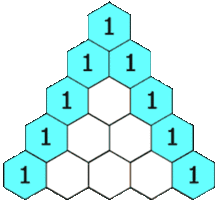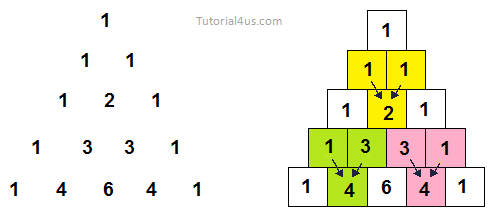# Print Pascals Triangle in Java

## Pascal Triangle Program in Java

First we know about Pascal Triangle what is this and how we design this triangle in general;
Pascal's triangle is a set of numbers arranged in the form of a triangle. Each number in a row is the sum of the left number and right number on the above row. If a number is missing in the above row, it is assumed to be 0. The first row starts with number 1. The following is a Pascal triangle with 5 rows.

Pascal's triangle is a triangular array of the binomial coefficients.## Java Program to Print Pascal Triangle

```import java.util.Scanner;
class Pascal
{
public static void main(String[] args)
{
int bin,p,q,r,x;
Scanner s=new Scanner(System.in);
System.out.println("How Many Row Do you want to input: ");
r=s.nextInt();
bin=1;
q=0;

System.out.print("Pascal's Triangle: ");

while(q<r)
{
for(p=40-3*q;p>0;--p)
System.out.print(" ");
for(x=0;x<=q;++x)
{
if((x==0)||(q==0))
bin=1;
else
bin=(bin*(q-x+1))/x;
System.out.print("      ");
System.out.print(bin);
}

System.out.println("");
++q;
}
}
}
```

## Output

```How Many Row Do you want to input:
5

1
1    1
1   2    1
1   3   3    1
1   4   6   4   1

```

## Syntax

```for compile -> c:/>javac Pascal.java
for run -> c:/>java Pascal
```

### Explanation of Code

• Scanner s=new Scanner(System.in): are used for receive input from keyboard.
• nextInt(): method are used for get integer type value from keyboard.
• System.out.println("....."): are used for display message on screen or console.
• System.out.print("....."): are used for display message on screen or console but curson not move in new line.
• System.out.println("....."): are used for display message on screen or console after that cursor move in new line due to println().

## Pure VPN Privide Lowest Price VPN Just @ \$1.65. Per Month with Non Detected IP Lowest Price Non Detected IP VPN